## Pages

Showing posts with label maximum. Show all posts
Showing posts with label maximum. Show all posts

### Interactive: Fermat's Theorem

Fermat's Theorem: If $$f$$ is a local maximum or minimum at $$c$$, and if $$f'(c)$$ exists, then $$f'(c)=0$$.

Critical Number: A number $$c$$ in the domain of $$f$$ such that either $$f'(c)=0$$ or $$f'(c)$$ does not exist.

Instructions: With the mouse, move point P along the function and you will see it's derivative traced in green. Here c and d are the critical numbers for the function graphed in blue. Refresh browser to start over.

### Intermediate Algebra Exam #3

Click on the 10 question exam covering topics in chapter 6. Give yourself one hour to try all of the problems and then come back and check your answers.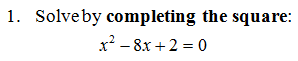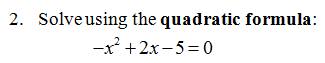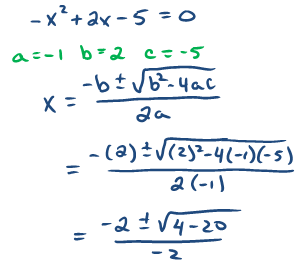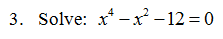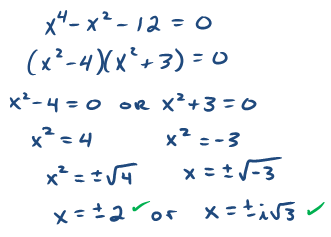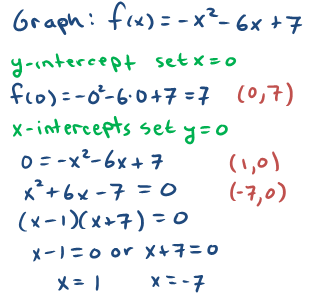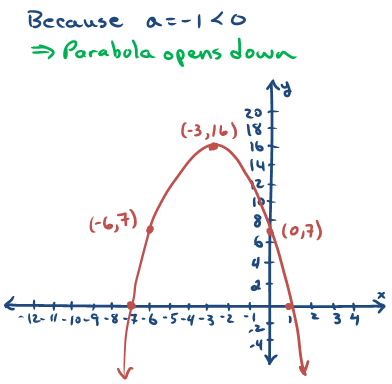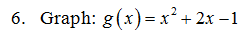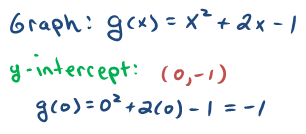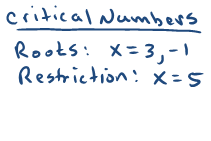9. The height of a baseball in feet tossed upward is given by the functionwhere t represents time in seconds.  What is the maximum height of the baseball?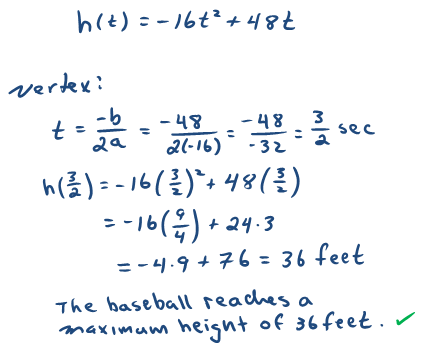10. Find a quadratic equation with solutions: {±5i}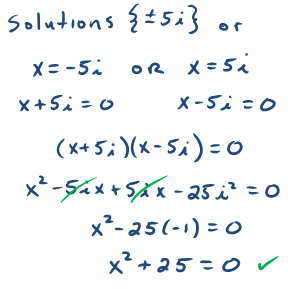---